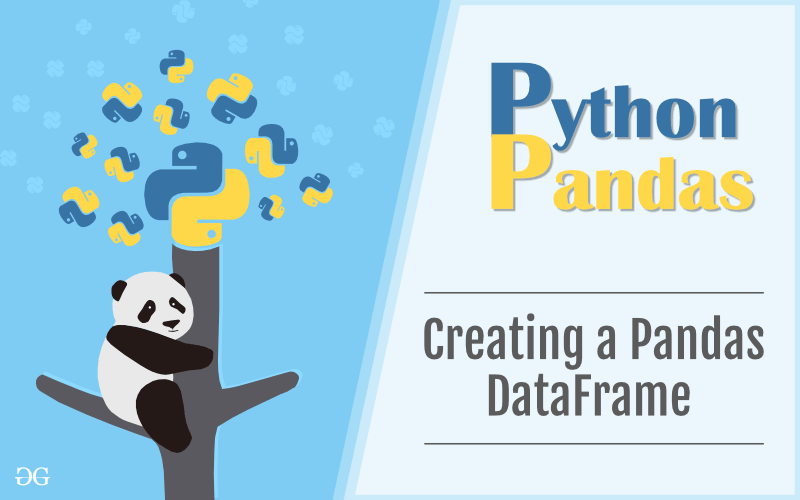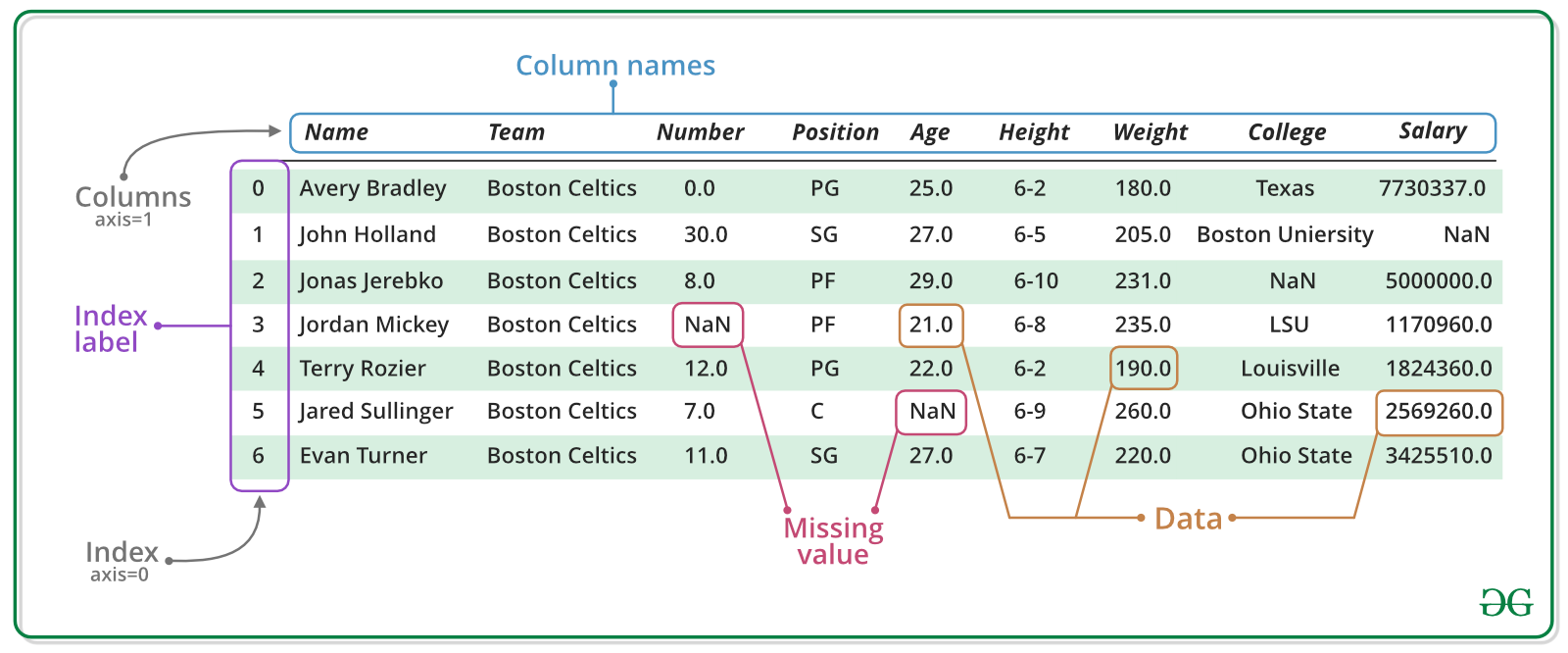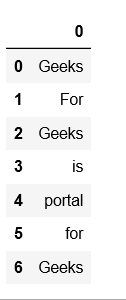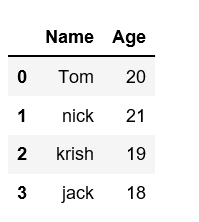# Creating a Pandas DataFrame

In the real world, a Pandas DataFrame will be created by loading the datasets from existing storage, storage can be SQL Database, CSV file, and Excel file. Pandas DataFrame can be created from the lists, dictionary, and from a list of dictionary etc.A Dataframe is a two-dimensional data structure, i.e., data is aligned in a tabular fashion in rows and columns. In dataframe datasets arrange in rows and columns, we can store any number of datasets in a dataframe. We can perform many operations on these datasets like arithmetic operation, columns/rows selection, columns/rows addition etc.Pandas DataFrame can be created in multiple ways. Let’s discuss different ways to create a DataFrame one by one.
Creating an empty dataframe :
A basic DataFrame, which can be created is an Empty Dataframe. An Empty Dataframe is created just by calling a dataframe constructor.

## Python3

 `# import pandas as pd` `import` `pandas as pd`   `# Calling DataFrame constructor` `df ``=` `pd.DataFrame()`   `print``(df)`

Output :

```Empty DataFrame
Columns: []
Index: []```

Creating a dataframe using List:
DataFrame can be created using a single list or a list of lists.

## Python3

 `# import pandas as pd` `import` `pandas as pd`   `# list of strings` `lst ``=` `[``'Geeks'``, ``'For'``, ``'Geeks'``, ``'is'``, ` `            ``'portal'``, ``'for'``, ``'Geeks'``]`   `# Calling DataFrame constructor on list` `df ``=` `pd.DataFrame(lst)` `print``(df)`

Output:Creating DataFrame from dict of ndarray/lists:
To create DataFrame from dict of narray/list, all the narray must be of same length. If index is passed then the length index should be equal to the length of arrays. If no index is passed, then by default, index will be range(n) where n is the array length.

## Python3

 `# Python code demonstrate creating ` `# DataFrame from dict narray / lists ` `# By default addresses.`   `import` `pandas as pd`   `# initialise data of lists.` `data ``=` `{``'Name'``:[``'Tom'``, ``'nick'``, ``'krish'``, ``'jack'``], ``'Age'``:[``20``, ``21``, ``19``, ``18``]}`   `# Create DataFrame` `df ``=` `pd.DataFrame(data)`   `# Print the output.` `print``(df)`

Output:Create pandas dataframe from lists using dictionary:
Creating pandas data-frame from lists using dictionary can be achieved in different ways. We can create pandas dataframe from lists using dictionary using pandas.DataFrame. With this method in Pandas we can transform a dictionary of list to a dataframe.

## Python3

 `# importing pandas as pd` `import` `pandas as pd`   `# dictionary of lists` `dict` `=` `{``'name'``:[``"aparna"``, ``"pankaj"``, ``"sudhir"``, ``"Geeku"``],` `        ``'degree'``: [``"MBA"``, ``"BCA"``, ``"M.Tech"``, ``"MBA"``],` `        ``'score'``:[``90``, ``40``, ``80``, ``98``]}`   `df ``=` `pd.DataFrame(``dict``)`   `print``(df)`

Output:Multiple ways of creating dataframe :

Whether you're preparing for your first job interview or aiming to upskill in this ever-evolving tech landscape, GeeksforGeeks Courses are your key to success. We provide top-quality content at affordable prices, all geared towards accelerating your growth in a time-bound manner. Join the millions we've already empowered, and we're here to do the same for you. Don't miss out - check it out now!